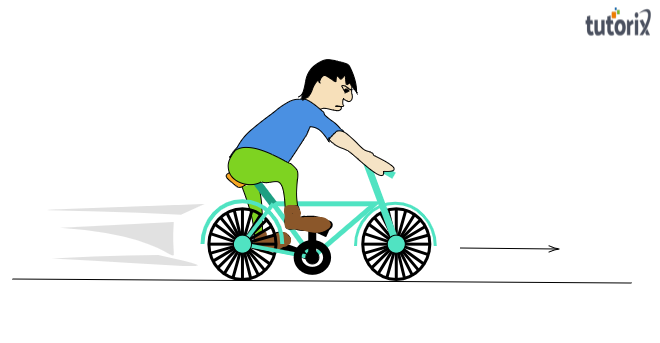# What are the various energy transformations that occur when you are riding a bicycle?

To do:
To write the various energy transformations that occur when someone is riding a bicycle.

Solution:
Before setting out the various energy changes that occur while cycling, let us briefly understand the motion of a bicycle:

Movement of a bicycle:
When a cyclist is riding a bicycle, the cyclist exerts a muscular force on the pedals of the bicycle and the wheels start rotating and the cycle moves forward.Now let us discuss the energy transformations that occur during the moment of a bicycle.

Energy transformations occurring while riding a bicycle:
From the movement of a bicycle, we got to understand that a bicycle moves forward when muscular force is exerted on its pedal. So, the muscular energy of the rider gets converted into the following form-

1. Heat energy
The heat energy heats the rider's body, making the rider tired and sweaty.
2. Kinetic energy of the bicycle
Kinetic energy provides a velocity for the bicycle to move forward.

This energy transformation can be shown below:
$\boxed{Muscular\ energy=kinetic\ energy+heat\ energy}$